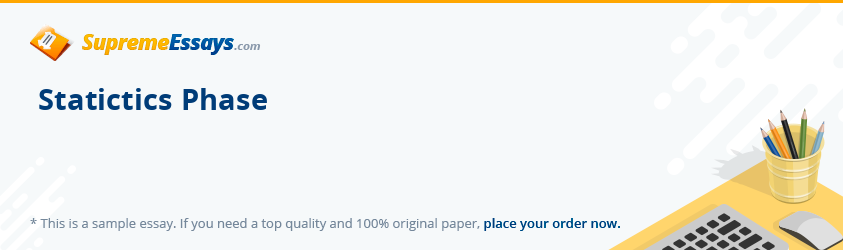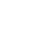Live chatDue to the direction that the company was taking, the CEO needed to have an analysis of the confidence level of their customers. There has been an anticipation to have at least a 95 % confidence level which is an achievable goal. In this case, the target for customer satisfaction was set at 4.5. Additionally, a sample of 20 adults was selected for which the average score was computed to 4.7 for which the standard deviation of this computation came to 0.25. Using the t test with the assumption that there is a normal distribution, the following results are obtained.

To be able to compute the t test score, the following formula is applied.

Such that t is the t-score, x is the sample mean; X is the population mean; S is the standard deviation of the sample; while n is the total number of members under consideration.

To calculate the degree of freedom, take n-1. In the sample under consideration the following is evident;

t = 0.24148408

A simulation of these results in the execution of the following data set.

Null hypothesis: population mean = 4.7

Sample size: n = 20

Sample mean = 4.5, std. deviation = 0.25

Test statistic: t (19) = (4.5 - 4.7)/0.0559017 = -3.57771

Two-tailed p-value = 0.002008

(One-tailed = 0.001004)

Based on the alternative hypothesis, this is a two-tailed test since the one-tailed test tends more towards the zero point than the two-tailed test. When this is run with the null hypothesis set at zero, the following results are evident.

Null hypothesis: Difference of means = 0

Sample 1:

n = 10, mean = 62600, s. d. = 33103.2

Standard error of mean = 10468.2

95% confidence interval for mean: 38919.4 to 86280.6

Sample 2:

Stay Connectedn = 10, mean = 3.7, s. d. = 1.41814

Standard error of mean = 0.448454

95% confidence interval for mean: 2.68553 to 4.71447

Test statistic: t (18) = (62600 - 3.7)/10468.2 = 5.97969

Two-tailed p-value = 1.175 e-005

(One-tailed = 5.874 e-006)

Taking an average of the test statistics yields 1.20099 which is much closer to the right tail probability given by P (Z>=x) that is closer to the two-tailed p-value. This implies that the most likely score for customer satisfaction will be 4.7787 that are above the set expectation by the CEO.

Coefficient of Correlation

Coefficient of correlation is a measure that is used to determine the strength in any linear relationship coupled with an evaluation of the direction for which this relation will take while comparing two variables. Coefficient of correlation is represented mathematically as follows.

Coefficient of correlation is significant in the sense that it gives the extent to which correlation coefficient, r, is unlikely to occur when there exists no significant relationship in the population under observation.

It is good to note that when the p-value is smaller, it means that the relationship is quite significant while larger p-values of the correlation indicate stronger relationships.

The following results are obtained upon calculation of coefficient of correlation on the data provided.

Corr (Income_1, SATISFACTION) = 0.91312794

Under the null hypothesis of no correlation:

t (8) = 6.33526, with two-tailed p-value 0.0002

Test for difference between Income_1 and SATISFACTION

Limited time Offer

0
0
days
:
0
0
hours
:
0
0
minutes
:
0
0
seconds
Get 19% OFF

Sign Test

Number of differences: n = 10

Number of cases with Income_1 > SATISFACTION: w = 10 (100.00%)

Under the null hypothesis of no difference, W follows B (10, 0.5)

Prob (W <= 10) = 1

Prob (W >= 10) = 0.000976563

From the results, it is clear that there is evidence of the fact that the most likely appearance is that there is significance in relationship. This means that there is likelihood that there will be high customer satisfaction score.

#### Related Accounting essays

0

Preparing Orders

0

Active Writers

0

Support Agents

Limited offerget 15% off your 1st order